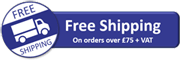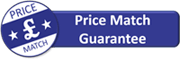# Rigol M301 Data Acquisition System

£1167.00 (£1400.40 inc VAT)

Available on back-order, delivery normally 1 - 2 weeks

Availability: Stock models delivered next working day (UK only) for orders placed before 15:00 (Mon - Fri)SKU: M301

## Rigol M301 Data Acquisition System

Rigol M300 series data acquisition systems are a cost effective solution for situations that require the automated monitoring of repetitive measurements (e.g. voltage, current, resistance, frequency, period, temperature & sensor) from various points within a circuit, assembly or system and the logging of results over a period of time. In product design and development environments, the Rigol M300 is ideal for design validation tests, accelerated life tests, burn-in tests and production tests. Furthermore, the various standard communications interfaces and alarm outputs allows the Rigol M300 to be controlled and monitored remotely. Hence it is also ideal as a basis for cost effective 24/7 remote process and/or system monitoring.

The Rigol M300 has 5-off slots that support any combination of 8-off types of plug-in modules: A DMM module, multiplexers, matrix switches and a multi-function module.

The M300 has a 4.3″ high-resolution colour LCD, user-friendly interface design, easy-to-use keyboard layout as well as clear backlit keys and operation prompts. The unit can be controlled remotely via any of its standard communications interfaces – RS232, USB, LAN and GPIB.

Each M300 system includes Rigol Ultra Acquire PC software as standard. Rigol Ultra Acquire Pro PC software and the powerful and flexible Multi-Channel Process System (MCPS) PC software from CAD-Computer are available as options.

The Rigol M301 is simply a package consisting of the Rigol M300 data acquisition / switch system and the Rigol MC3065 DMM module. Buying this combination as the Rigol M301 offers a considerable saving over buying these items separately.

## Documentation

#### User Manual

DC Characteristics

Function Range Test Current or Load Voltage 24 Hour ℃±1℃ 90 Day ℃±5℃ 1 Year ℃ ±5℃ Temperature Coefficient 0℃ to (TCAL℃ -5℃ ) (TCAL℃ +5℃ ) to 50℃
DC Voltage 200.0000mV
2.000000V
20.00000V
200.0000V
300.000V

0.0020 + 0.0020
0.0015 + 0.0005
0.0020 + 0.0004
0.0020 + 0.0006
0.0020 + 0.0006
0.0030 + 0.0025
0.0020 + 0.0006
0.0030 + 0.0005
0.0040 + 0.0006
0.0040 + 0.0010
0.0040 + 0.0025
0.0035 + 0.0006
0.0040 + 0.0005
0.0050 + 0.0006
0.0055 + 0.0010
0.0005 + 0.0005
0.0005 + 0.0001
0.0005 + 0.0001
0.0005 + 0.0001
0.0005 + 0.0001
DC Current 200.0000µA
2.000000mA
20.00000mA
200.0000mA
1.000000A
<0.03V
<0.25V
<0.07V
<0.7V
<0.12V
0.010 + 0.012
0.007 + 0.003
0.007 + 0.012
0.010 + 0.002
0.050 + 0.020
0.040 + 0.015
0.030 + 0.003
0.030 + 0.015
0.030 + 0.003
0.080 + 0.020
0.050 + 0.015
0.050 + 0.003
0.050 + 0.015
0.050 + 0.003
0.100 + 0.020
0.0020 + 0.0030
0.0020 + 0.0005
0.0020 + 0.0020
0.0020 + 0.0005
0.0050 + 0.0010
Resistance 200.0000Ω
2.000000kΩ
20.00000kΩ
200.0000kΩ
1.000000MΩ
10.00000MΩ
100.0000MΩ
1mA
1mA
100µA
10µA
2µA
200nA
200nA || 10MΩ
0.0030 + 0.0030
0.0020 + 0.0005
0.0020 + 0.0005
0.0020 + 0.0005
0.002 + 0.001
0.015 + 0.001
0.300 + 0.010
0.008 + 0.004
0.008 + 0.001
0.008 + 0.001
0.008 + 0.001
0.010 + 0.001
0.030 + 0.001
0.800 + 0.010
0.010 + 0.004
0.010 + 0.001
0.010 + 0.001
0.010 + 0.001
0.012 + 0.001
0.040 + 0.001
0.800 + 0.010
0.0050 + 0.0010
0.0006 + 0.0001
0.0006 + 0.0001
0.0006 + 0.0001
0.0010 + 0.0002
0.0030 + 0.0004
0.1500 + 0.0002

AC Characteristics

Function Range Frequency Range 24 Hour ℃±1℃ 90 Day ℃±5℃ 1 Year ℃ ±5℃ Temperature Coefficient 0℃ to (TCAL℃ -5℃ ) (TCAL℃ +5℃ ) to 50℃
True RMS AC Voltage 200.0000mV 3Hz- 5Hz
5Hz-10Hz
10Hz-20kHz
20kHz-50kHz
50kHz-100kHz
100kHz- 300kHz
1.00 + 0.03
0.35 + 0.03
0.04 + 0.03
0.10 + 0.05
0.55 + 0.08
4.00 + 0.50
1.00 + 0.04
0.35 + 0.04
0.05 + 0.04
0.11 + 0.05
0.60 + 0.08
4.00 + 0.50
0.00 + 0.04
0.35 + 0.04
0.06 + 0.04
0.12 + 0.05
0.60 + 0.08
4.00 + 0.50
0.100 + 0.004
0.035 + 0.004
0.005 + 0.004
0.011 + 0.005
0.060 + 0.008
0.20 + 0.02
2.000000V 3Hz-5Hz
5Hz-10Hz
10Hz – 20kHz
20kHz – 50kHz
50kHz – 100kHz
100kHz – 300kHz
1.00 + 0.02
0.35 + 0.02
0.04 + 0.02
0.10 + 0.04
0.55 + 0.08
4.00 + 0.50
1.00 + 0.03
0.35 + 0.03
0.05 + 0.03
0.11 + 0.05
0.60 + 0.08
4.00 + 0.50
1.00 + 0.03
0.35 + 0.03
0.06 + 0.03
0.12 + 0.05
0.60 + 0.08
4.00 + 0.50
0.100 + 0.003
0.035 + 0.003
0.005 + 0.003
0.011 + 0.005
0.060 + 0.008
0.20 + 0.02
20.00000V 3Hz-5Hz
5Hz – 10Hz
10Hz – 20kHz
20kHz – 50kHz
50kHz – 100kHz
100kHz – 300kHz
1.00 + 0.03
0.35 + 0.03
0.04 + 0.04
0.10 + 0.05
0.55 + 0.08
4.00 + 0.50
1.00 + 0.04
0.35 + 0.04
0.07 + 0.04
0.12 + 0.05
0.60 + 0.08
4.00 + 0.50
1.00 + 0.04
0.35 + 0.04
0.08 + 0.04
0.15 + 0.05
0.60 + 0.08
4.00 + 0.50
0.100 + 0.004
0.035 + 0.004
0.008 + 0.004
0.012 + 0.005
0.060 + 0.008
0.20 + 0.02
200.0000V 3Hz-5Hz
5Hz-10Hz
10Hz-20kHz
20kHz-50kHz
50kHz-100kHz
100kHz-300kHz
1.00 + 0.02
0.35 + 0.02
0.04 + 0.02
0.10 + 0.04
0.55 + 0.08
4.0 + 0.50
1.00 + 0.03
0.35 + 0.03
0.07 + 0.03
0.12 + 0.05
0.60 + 0.08
4.0 + 0.50
1.00 + 0.03
0.35 + 0.03
0.08 + 0.03
0.15 + 0.05
0.60 + 0.08
4.0 + 0.50
0.100 + 0.003
0.035 + 0.003
0.008 + 0.003
0.012 + 0.005
0.060 + 0.008
0.20 + 0.02
300.000V 3Hz-5Hz
5Hz-10Hz
10Hz-20kHz
20kHz-50kHz
50kHz-100kHz
100kHz-300kHz
1.00 + 0.02
0.35 + 0.02
0.04 + 0.02
0.10 + 0.04
0.55 + 0.08
4.0 + 0.50
1.00 + 0.03
0.35 + 0.03
0.07 + 0.03
0.12 + 0.05
0.60 + 0.08
4.0 + 0.50
1.00 + 0.03
0.35 + 0.03
0.08 + 0.03
0.15 + 0.05
0.60 + 0.08
4.0 + 0.50
0.100 + 0.003
0.035 + 0.003
0.008 + 0.003
0.012 + 0.005
0.060 + 0.008
0.20 + 0.02
True RMS AC Current 200.0000µA 3Hz-5Hz
5Hz-10Hz
10Hz-5kHz
5kHz-10kHz
1.10 + 0.06
0.35 + 0.06
0.15 + 0.06
0.35 + 0.70
1.10 + 0.06
0.35 + 0.06
0.15 + 0.06
0.35 + 0.70
1.10 + 0.06
0.35 + 0.06
0.15 + 0.06
0.35 + 0.70
0.200 + 0.006
0.100 + 0.006
0.015 + 0.006
0.30 + 0.006
2.000000mA 3Hz-5Hz
5Hz-10Hz
10Hz-5kHz
5kHz-10kHz
1.00 + 0.04
0.30 + 0.04
0.12 + 0.04
0.20 + 0.25
1.00 + 0.04
0.30 + 0.04
0.12 + 0.04
0.20 + 0.25
1.00 + 0.04
0.30 + 0.04
0.12 + 0.04
0.20 + 0.25
0.100 + 0.006
0.035 + 0.006
0.015 + 0.006
0.030 + 0.006
20.00000mA 3Hz-5Hz
5Hz-10Hz
10Hz-5kHz
5kHz-10kHz
1.10 + 0.06
0.35 + 0.06
0.15 + 0.06
0.35 + 0.70
1.10 + 0.06
0.35 + 0.06
0.15 + 0.06
0.35 + 0.70
1.10 + 0.06
0.35 + 0.06
0.15 + 0.06
0.35 + 0.70
0.200 + 0.006
0.100 + 0.006
0.015 + 0.006
0.30 + 0.006
200.0000mA 3Hz-5Hz
5Hz-10Hz
10Hz-5kHz
5kHz-10kHz
1.00 + 0.04
0.30 + 0.04
0.10 + 0.04
0.20 + 0.25
1.00 + 0.04
0.30 + 0.04
0.10 + 0.04
0.20 + 0.25
1.00 + 0.04
0.30 + 0.04
0.10 + 0.04
0.20 + 0.25
0.100 + 0.006
0.035+0.006
0.15+0.006
0.30+0.006
1.000000A 3Hz-5Hz
5Hz-10Hz
10Hz-5kHz
5kHz-10kHz
1.10 + 0.06
0.35 + 0.06
0.15 + 0.06
0.35 + 0.70
0.35 + 0.08
0.15 + 0.08
1.10 + 0.06
0.35 + 0.06
0.15 + 0.06
0.35 + 0.70
0.35 + 0.10
0.15 + 0.10
1.10+0.06
0.35+0.06
0.15+0.06
0.35+0.70
0.35+0.10
0.15+0.10
0.100+0.006
0.035+0.006
0.015+0.006
0.030+0.006
0.035+0.008
0.015+0.008

Note*

Specifications are liable to change. For the full list of Specifications, please see the products datasheet located under the ‘Documentation’ tab.

## Reviews

There are no reviews yet.

Only logged in customers who have purchased this product may leave a review.